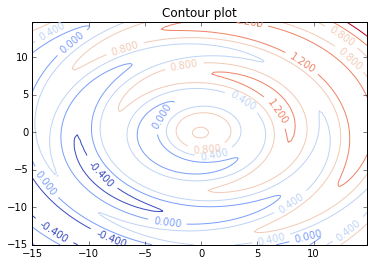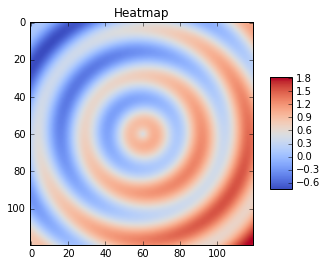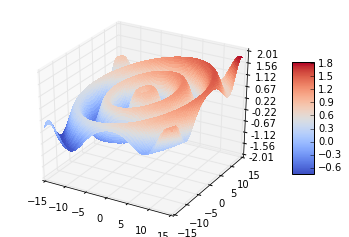## Plotting functions of two variables¶

This is basically a subset of this tutorial. Here is how we can produce a surface plot:

In :
#Needed to display images inline in Jupyter
%matplotlib inline
####################################

from mpl_toolkits.mplot3d import Axes3D
from matplotlib import cm
from matplotlib.ticker import LinearLocator, FormatStrFormatter
from matplotlib.pyplot import *
from numpy import *


Let's first draw a contour plot -- the contours are lines such that $f(x, y) = c$ for some $c$.

In :
x = arange(-15, 15, 0.25)
y = arange(-15, 15, 0.25)
X, Y = meshgrid(x, y)
R = sqrt(X**2 + Y**2)
Z = -.4 + (X+15)/30. + (Y+15)/40.+.5*sin(R)

figure(1)
CS = contour(X, Y, Z, cmap=cm.coolwarm)
clabel(CS, inline=1, fontsize=10)
title('Contour plot')

show()Now, let's display a heatmap. You can see the contours on the heatmap.

In :
fig = figure(2)
ax = fig.gca()
heatmap = ax.imshow(Z, cmap = cm.coolwarm)
fig.colorbar(heatmap, shrink = 0.5, aspect=5)
title('Heatmap')
show()Finally, here is a surface plot. The heatmap is just the surface plot, looked at from above (assuming that the colours are also used on the surface, of course.)

In :
fig = figure(3)
ax = fig.gca(projection='3d')
surf = ax.plot_surface(X, Y, Z, rstride=1, cstride=1, cmap=cm.coolwarm,
linewidth=0, antialiased=False)
ax.set_zlim(-2.01, 2.01)

ax.zaxis.set_major_locator(LinearLocator(10))
ax.zaxis.set_major_formatter(FormatStrFormatter('%.02f'))

fig.colorbar(surf, shrink=0.5, aspect=5)
show()